## Square Root Method

The square root method is an algorithm which solves the Matrix Equation(1)

for, withaSymmetric Matrix and g a given Vector. Convertto a Triangular Matrix such that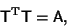(2)

whereis the Matrix Transpose. Then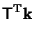(3)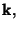(4)

so(5)

giving the equations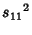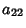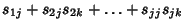(6)

These give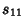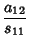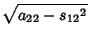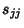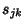(7)

giving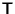from A. Now solve for k in terms of thes and g,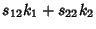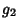(8)

which gives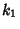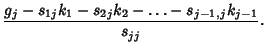(9)

Finally, findfrom thes and k,(10)

giving the desired solution,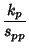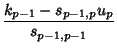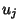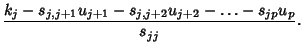(11)